# [阅读总结]PointCloud Basic Architecture总结梳理

## 对自己阅读的五种论文进行一下总结，也在总结中对于点云处理的基础深度学习框架有了透彻的理解，不得不说写出这些文章的真的好天才啊，星星眼！

Posted by Xuehui Wang on 2019-07-13

# PointNet

## contribution：

1. 设计了一个可以应对无序3D点云的深度学习网络框架PointNet
2. 使用MLP作为连续函数的离散化模拟以及max pooling作为对称函数应对点云输入的置换不变性，二者的结合在理论上可以获得任意连续函数。
3. 提出了转换网络来应对刚体变换等。

## 做法：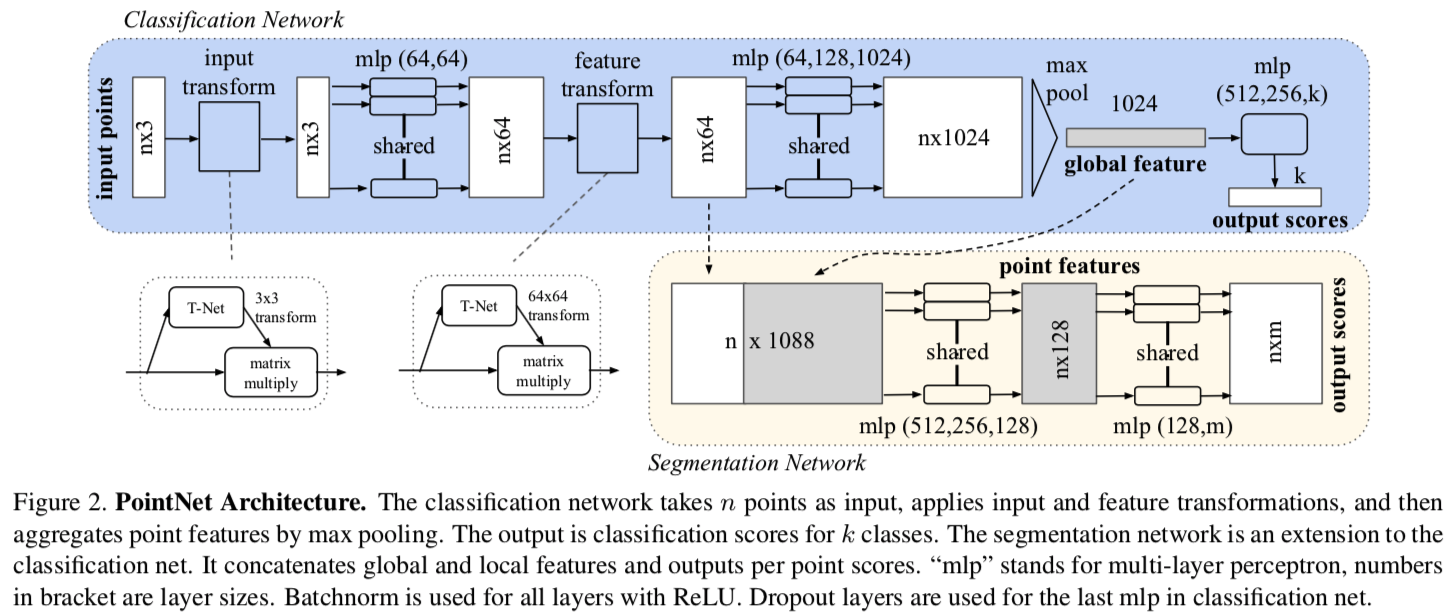1.输入就是$n$个点，每个点有3个坐标作为特征，这样整体的输入就是$n \times 3$

2.首先将输入点云送到输入变换网络中，这一步是学习一个$3 \times 3$的变换网络，将输入点云进行校准，在于旋转不变性。然后获得旋转角度校准后的$n \times 3$的矩阵。

3.然后使用MLP进行特征的提取，MLP是通过共享权重的卷积实现的，并不是全连接，从每个点3维特征提升到每个点64维，因此得到了$n \times 64$的特征输出，同时记录下来。作者认为这个可以作为Point-wise特征

4.（可选）同样的将点云映射到64维的冗余空间后，再对64维的点云特征做一次校对，只不过这次校对需要引入一个正则化惩罚项，希望其尽可能接近于一个正交矩阵

5.随后使用MLP进行连续两次特征提取，映射到1024为空间，得到$n \times 1024$的输出，在使用max pooling进行整合，得到一个1024大小的向量，这就是全局特征

6.在分类任务中，这1024维向量通过全连接得到一个$k$维向量，加softmax，得到k个类别的预测程度。

7.在分割任务中，作者把1024维的向量完全复制为$n \times 1024$,之后和之前记录的Point-wise特征$n \times 64$进行拼合得到$n \times 1088$。作者认为这样就是一个全局+Point-wise的综合特征，可以用来做分割。

8.分割网络是将$n \times 1088$使用MLP提取得到$n \times 128$的特征，再用MLP提取得到$n \times m$的特征，m是语义分割的种类数，最后经过soft_max这样就是n个点，每个点有m种对应的种类。取最大即为对一个点的预测。

## 实验结果

accuracy avg.class accuracy overall
PointNet 86.2 89.2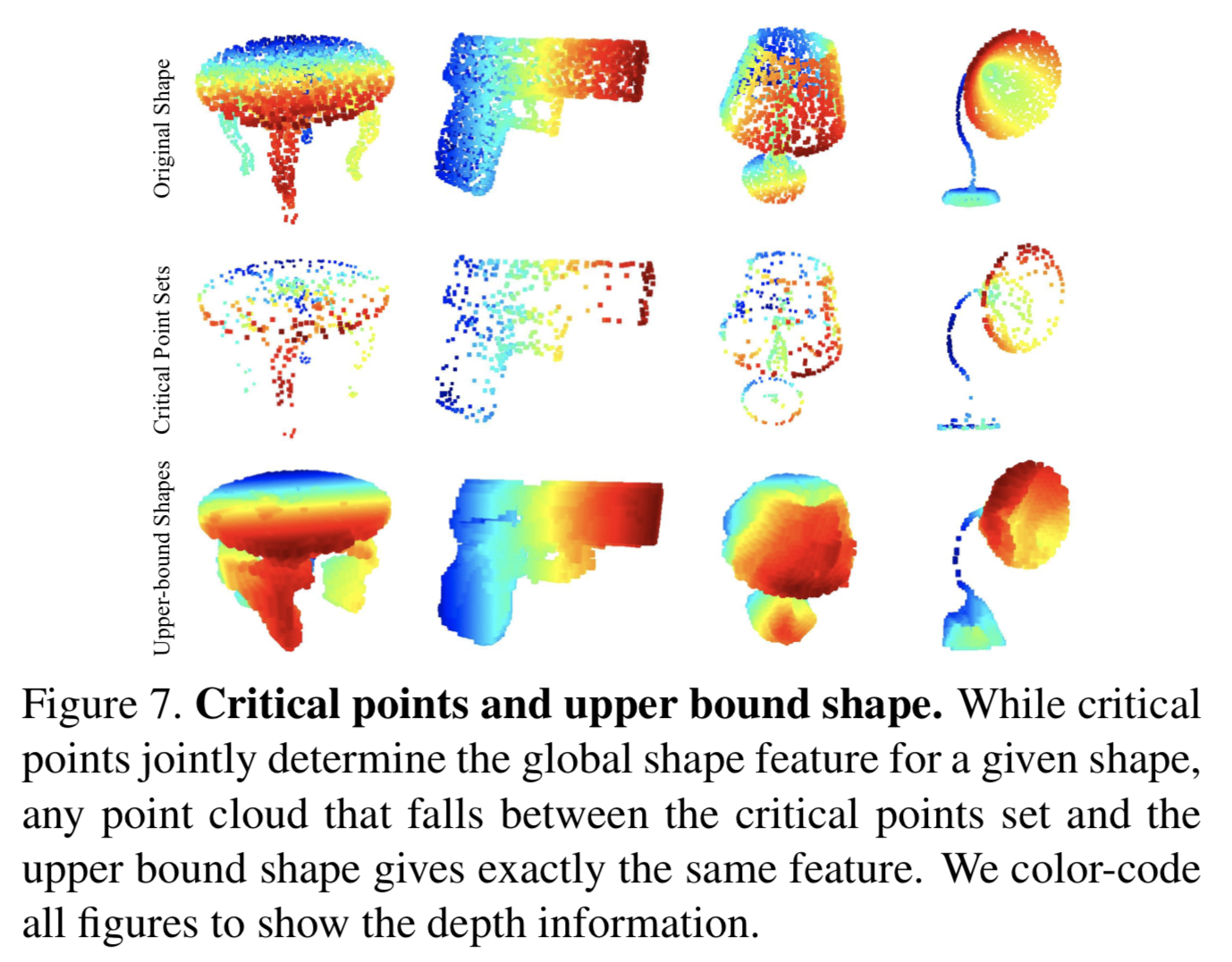# PointNet++

## contributions：

• 是一种分层网络。它可以将PointNet网络递归的在嵌套分隔的点云集上使用，通过对度量空间距离的使用，可以学习带有上下文尺度信息的局部特征，并使得越到后边的特征图感受野越大。
• 同时还注意到了点集经常是不同密度的抽样结果，这种方式大幅度的降低了网络在统一密度点集上的训练效果，因此也提出了一种先进的集合学习层(set learning layer)来自适应的结合不同尺度的特征。

## 做法：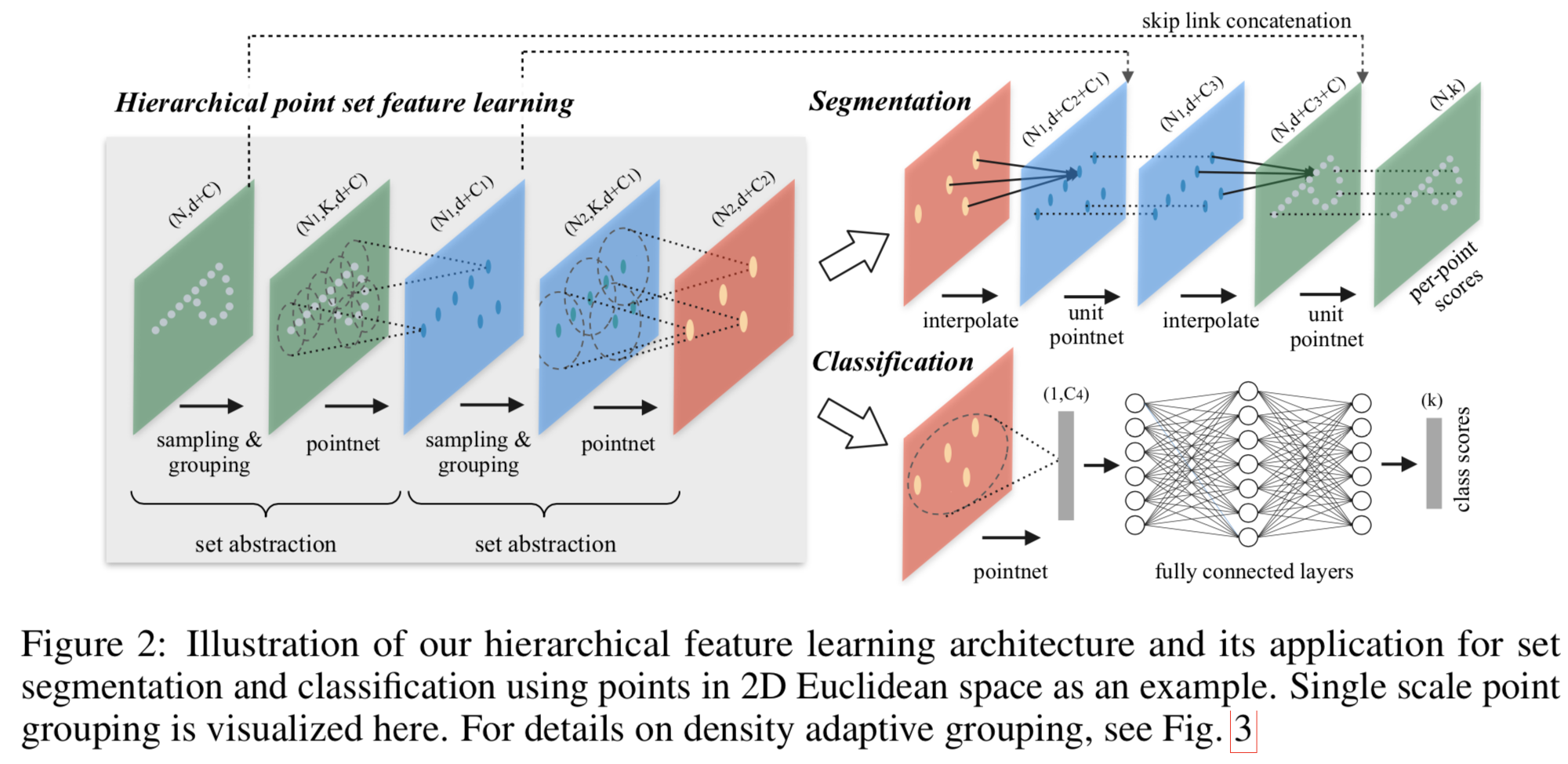• Sampling：利用FPS（最远点采样）随机采样点
• Grouping：利用Ball Query划一个R为半径的圈，将每个圈里面的点云作为一簇
• PointNet： 对Sampling+Grouping以后的点云进行局部的全局特征提取

### 分层特征学习

• 通过使用PointNet，将其作为一个特征提取器，使用分层的方式来迭代抽象出越来越大的区域

• 分层结构的主要组成部分是一堆集合抽象层(set abstraction layers)，处理 $N \times (d + C)$ 的矩阵，其中$N$是点云数量，$d$是每个点的坐标系维度，$C$是额外的点的特征维度（比如颜色，法向量，亮度）,输出$N’ \times (d+C’)$ 的矩阵，其中$N’$是经过该层下采样后得到的点云数量，$C’$是新的能概括局部信息的特征维度，每一层中的点都被抽象为下一层更少的点，就是卷积那种赶脚。一个抽象层分为三部分：

• 采样层（Sampling）-从点集中选择出一个子集，子集中每一个点都是一个局部区域的中心

使用FPS方法得到一个点子集，每一个点都是距离子集中其他点最远的点。相比随机采样这种方法的收敛性更强，与CNN扫描（感受野）分布未知的向量空间相比，这种采样策略的感受野范围与数据是相互独立的，也就是不用在意数据的量。

• 分组层（Grouping）-对子集中的每一个点搜索他们的近邻点，这样就组成了一个个局部区域。可能相互之间会有重叠(overlap)

输入是$N \times (d + C)$ 的数据和大小为$N’ \times d$ 的中心点的坐标($N’$应该就是子集的数量，$d$是每个中心点的坐标系维度)。输出是一组组的点集，大小为$N’ \times K \times (d+C)$,其中$K$是每一个中心点邻域范围内的点的数量，每一组对应一个局部区域，$K$在不同组中可能是不同的，但是不影响，因为PointNet模块对于输入数量没啥限制，反正都是输出一个特征向量。

在卷积中，图像的局部区域是通过kernel的size范围来确定的，在点云的抽样中，一个点的邻域范围是通过度量空间得到的。(也就是这一簇是以度量后进行的group)

分组方式是使用了球体选择(ball query)，也就是给定一个以中心点为体心的球，给半径，然后再这个半径组成的球体内取不超过$K_{max}$ 个点组成一组。另外有种代替方案是KNN，但是球体选择这种方案的好处就是得到的局部近邻保证了一个固定尺度的区域，这样使得这个局部区域的特征更加泛化，这对于局部识别任务（比如点云语义分割）更合适。

• PointNet层(算是特征提取层)-使用一个mini-PointNet对局部区域进行编码得到一个特征向量

对于分组层的输出，PointNet层将每一个group的中心点以及其周围的局部特征(从邻域点编码而来)进行抽象得到一个整体的局部区域，所以输出是$N’ \times (d+C’)$

在这一层中，进行抽象前首先将邻域点的坐标变成相对于中心点的相对坐标：$x_i^{(j)}=x_i^{(j)}-\hat{x}^{j}$ ，其中$i=1,2…,K$,$j=1,2…,d$ 。然后$\hat{x}$是中心点的坐标。这样之后就可以使用PointNet作为一个基础模块来对一个group进行抽象得到下一层的一个输入点。

### 对于非均匀采样密度的鲁棒性解决方案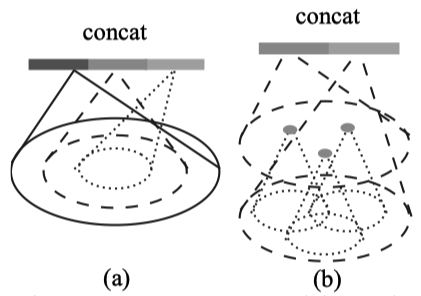a是multi-scale grouping，b是multi-resolution grouping
• 问题来源于点云在不同区域密度不同，即非均匀采样。在密集的区域中学到的特征可能对稀疏区域无法泛化，这样就会使得在稀疏点云中模型无法识别到精细的局部结构。
• 最理想的当然是在密集区域可以尽可能的得到最精细的细节，但在采样不足的区域就是天方夜谭。因此比较好的方法就是扩大采集的尺度。
• 所以本文提出了一种密度自适应的PointNet层，可以在输入的采样密度改变的时候学会结合区域多尺度下的特征，即是PointNet++。
• 在PointNet++的set abstraction layers中，会提取多个尺度的局部点，然后把他们结合起来。为了group局部区域以及结合多个尺度的特征，提出了MSG和MRG两种方案。
• Multi-scale grouping：最简单也有效的方式就是使用group layers来多次获得不同尺度的子集(也就是多个$N’$,但是每一个中心点对应有的$K$数量不同)，紧接着使用PointNet来提取多尺度子集的各自特征，然后把他们concat起来。形成了多尺度特征。
• Multi-resolution grouping：上边的方法是比较耗时的，因为对于每一种尺度PointNet layer都要对每个中心点进行处理一次。而中心点个数在特征层次比较低时数量是很多的，所以及其耗时。所以换一种又省时间，又可以根据点的分布特点来自适应的聚合信息的方法。即：在一些层$L_i$中，局部区域的特征是由两个特征拼合起来的。一个是对$L_{i-1}$层中几个中心点子集经过set abstraction layer得到的向量进行合并后的总特征向量，另一个是对这几个中心点集合的并集进行一起的PointNet得到的向量。比如在第1层中，1，2，3三个中心点集合在第2层中是一个集合，那第2层的输出特征就是1，2，3特征的汇总再加1，2，3三个集合所有点一起送入PointNet后得到的特征。 在点云稀疏时，第一个特征的依赖程度(或者说权重)应该低于第二个特征。因为第一个特征在计算时候点云很稀疏，抽样能力降低。而第二个特征因为是几个点集一起算，相当于在稀疏时扩大了区域。

### 分割任务

• 想解决的是在分割问题中，需要对每一个点都作出label的预测，但是set abstraction level是一种降采样。一种解决方式是将每一个点都当做一个中心点，但是这种方式对于计算资源的开销是巨大的。另一种方式是从降采样后的点中将特征传递到原始点集中。
• 大致流程：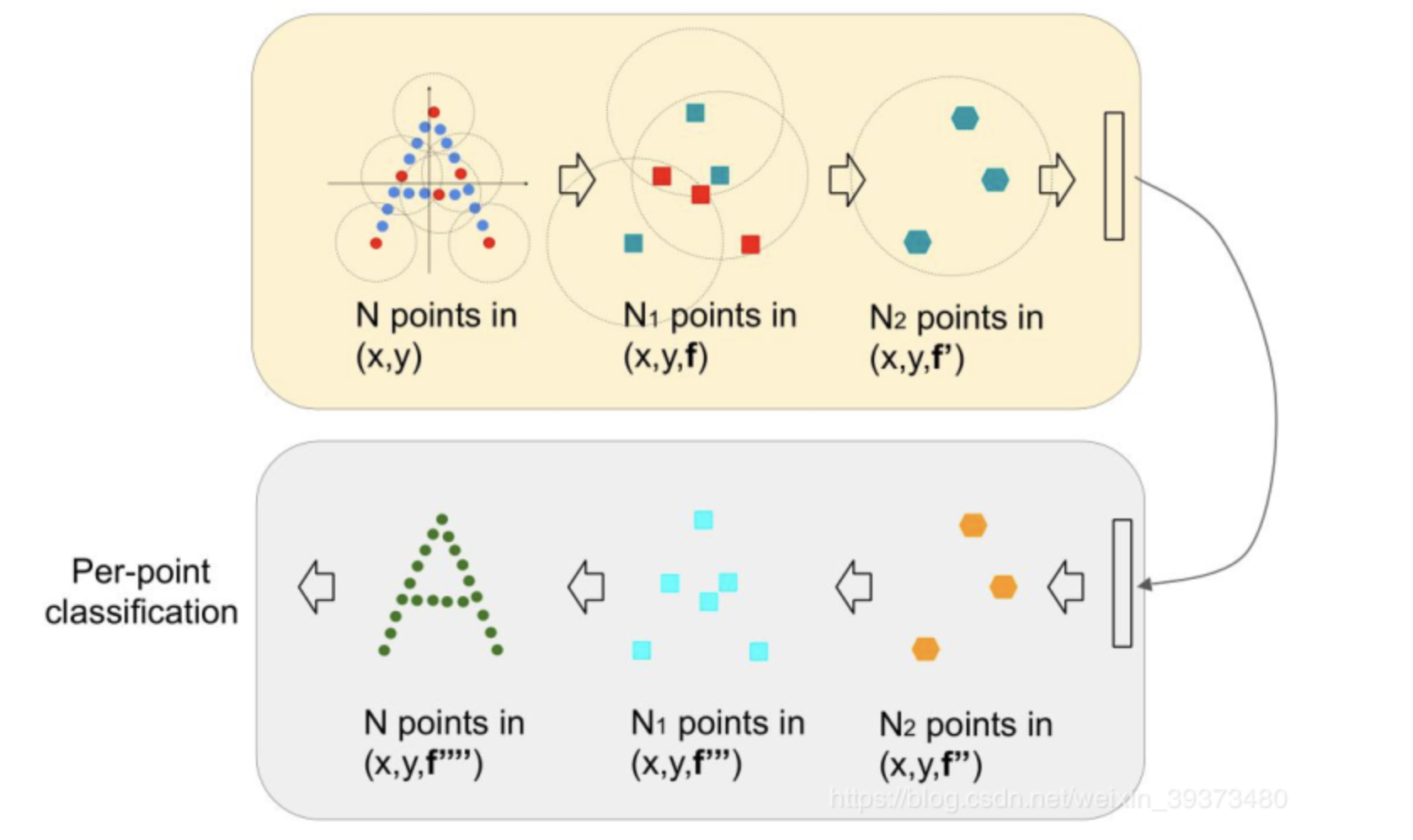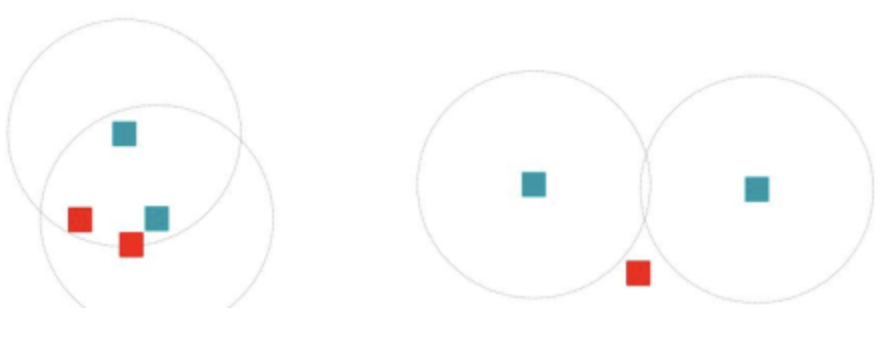## 实验结果

accuracy avg.class accuracy overall
PointNet++ - 90.7
PointNet++(with normal) - 91.9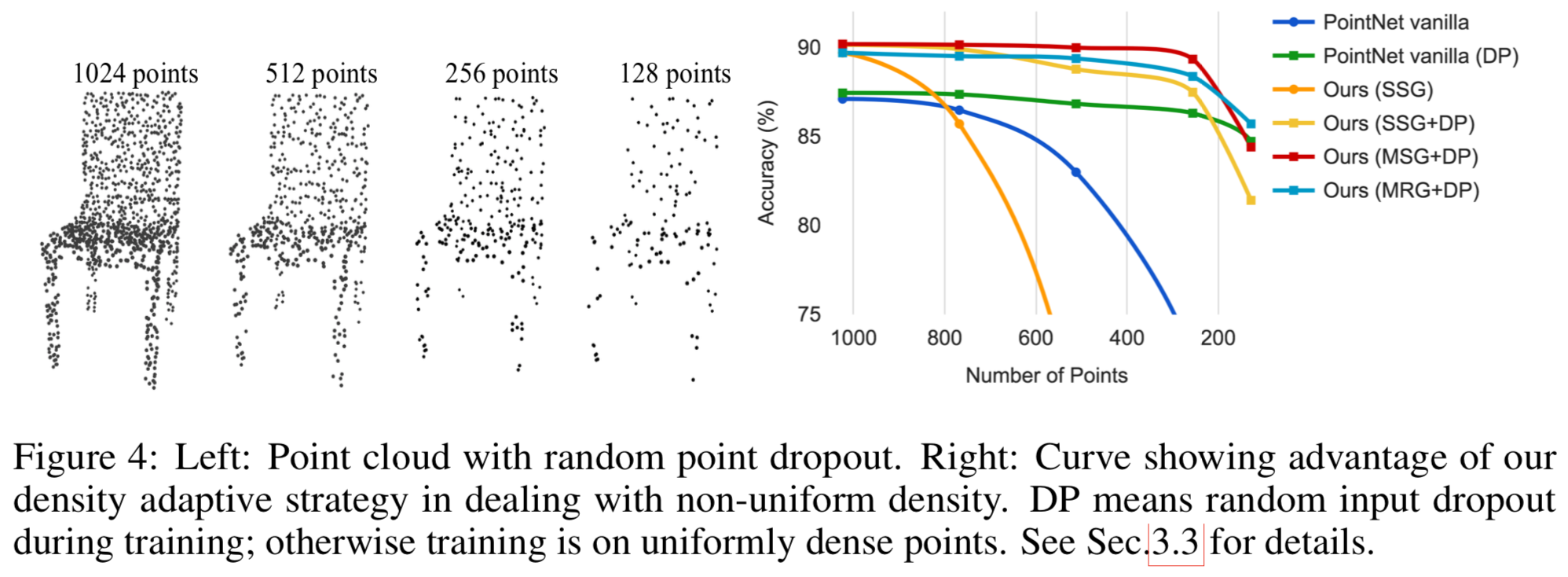# RS-CNN

## contribution：

• 提出了一个从关系中进行学习的卷积操作，即基于几何关系的卷积层。它可以对点云的几何关系进行编码。
• 基于以上提出了一个多层的几何关系卷积网络，命名为RS-CNN，可以将在2D中规则的卷积应用到不规则的空间中，以此来实现在点云上基于上下文的图形化学习。

## 做法：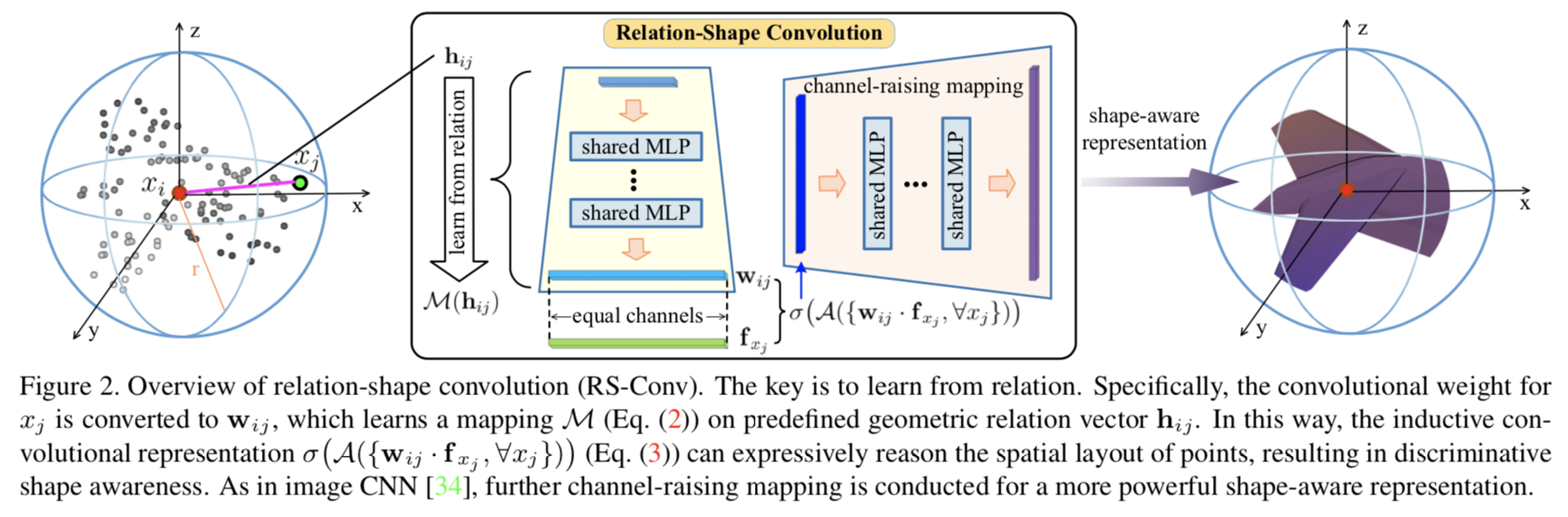1. 建模：对于一个球形的点云子集$P_{sub}$ ,需要学习到他的归纳性表达$f_{P_{sub}}$这样可以将潜在的形状信息编码成不同的特征。

其中，$\mathcal{N}(x_i)$是以$x_i$为中心点的一个点云子集，$d_{ij}$是表示点$xi$ 与$x_j$之间的欧氏距离。$r$是球体的半径，首先将所有$\mathcal{N}(x_i)$ 中所有点的特征使用$\mathcal{T}$进行变换，然后使用函数$\mathcal{A}$进行聚合，最后再通过一个非线性激活函数$\sigma$。为了保持平移不变性，这里的$\mathcal{A}$只能取对称函数。

2. 经典CNN中的局限性

在经典CNN中，$\mathcal{T}$通常的实现方法是$\mathcal{T}(x_j)=w_j \cdot f_{x_j}$。$w_j$是学习到的权重。

• $w_j$无法对每个点云都共享，这会导致对于平移变换的不变性丧失， 无法处理不规则的点云集合(即每个点云集合中点的数量是不变的)。
• 在反向传播过程中$w_j$的的梯度仅仅和独立的$x_j$有关，会导致一个未知的学习策略，即无法获得更多的形状意识与鲁棒性。该问题可以通过数据增强或者增加卷积核的数量减缓。
3. 作出改变：从关系中学习

• 作者认为以上的问题（局限性）可以从关系学习中解决。论文主张在点云中一个点$x_i$与其周围的点有一定的几何关系，并且这种关系是一种关于点云空间布局明确的表达，这可以有区别性的反应出一些潜在的形状。
• 因此在文中为了捕捉这种关系，他把卷积参数$w_i$换成了$w_{ij}$，这个值是通过对相关性特征$h_{ij}$加一个映射$\mathcal{M}$得到的，比如说关于$x_i$和$x_j$之间的预定义的几何先验。$h_{ij}$是一个低维的相关性特征。也就是说$\mathcal{T}(x_j)$变成了：

使用$\mathcal{M}$的目的就是将两点之间低层次的相关性特征进行提取，这样相当于对空间布局进行编码。在这篇论文中，这个$\mathcal{M}$使用MLP来实现的。同时，此时的$w_{ij}$在求导时也是和$w_i$与$w_j$都有关系。并且这个$M$也是可以对所有点进行共享的，保证了刚体变换的不变性。所以最开始的$f_{P_{sub}}$变成了：

这其实将$x_i$和他一个子集内的所有点的关系都连接了起来，可以生成有区别性的几何意识。

• 对于$h_{ij}$的确定（本质是一个几何先验），论文论证了多种方案，最基础的就是两点之间的欧距3D-Ed，也可以再加上其他维度，也取得了多个实验结果：
model low-level relation $h$ channels acc.
A (3D-Ed) 1 92.5
B (3D-Ed, $x_i-x_j$) 4 93.0
C (3D-Ed, $x_i-x_j, x_i, x_j$) 10 93.6
D (3D-cosd, $x^{nor}_i, x^{nor}_j$) 7 92.8
E (2D-Ed, $x’_i-x’_j, x’_i, x’_j$) 10 92.2
1. 总结

首先，选择合适的几何先验表达方式，论文里选择了上文的C方案，也就是一个$h_{ij}$是一个10通道的向量。

然后，使用MLP作为$\mathcal{M}$作为映射函数，将这种几何关系映射到高维空间。

其次，使用$\mathcal{A}$这个对称函数(比如max pooling，ave pooling)选出其中突出的相应

再然后，使用非线性激活函数输出最终一个子集的特征$f_{P_{sub}}$。这就融合了点与点之间的关系。

最后，可以用MLP将这个特征进行升维或者降维，用于不同的任务。

## 实验结果

accuracy avg.class accuracy overall
Relation-shape CNN - 93.6

# PointWeb

## contributions：

• 提出了一个PointWeb网络，关键模块就是AFA。并以此实现了较好的实验效果。

## 做法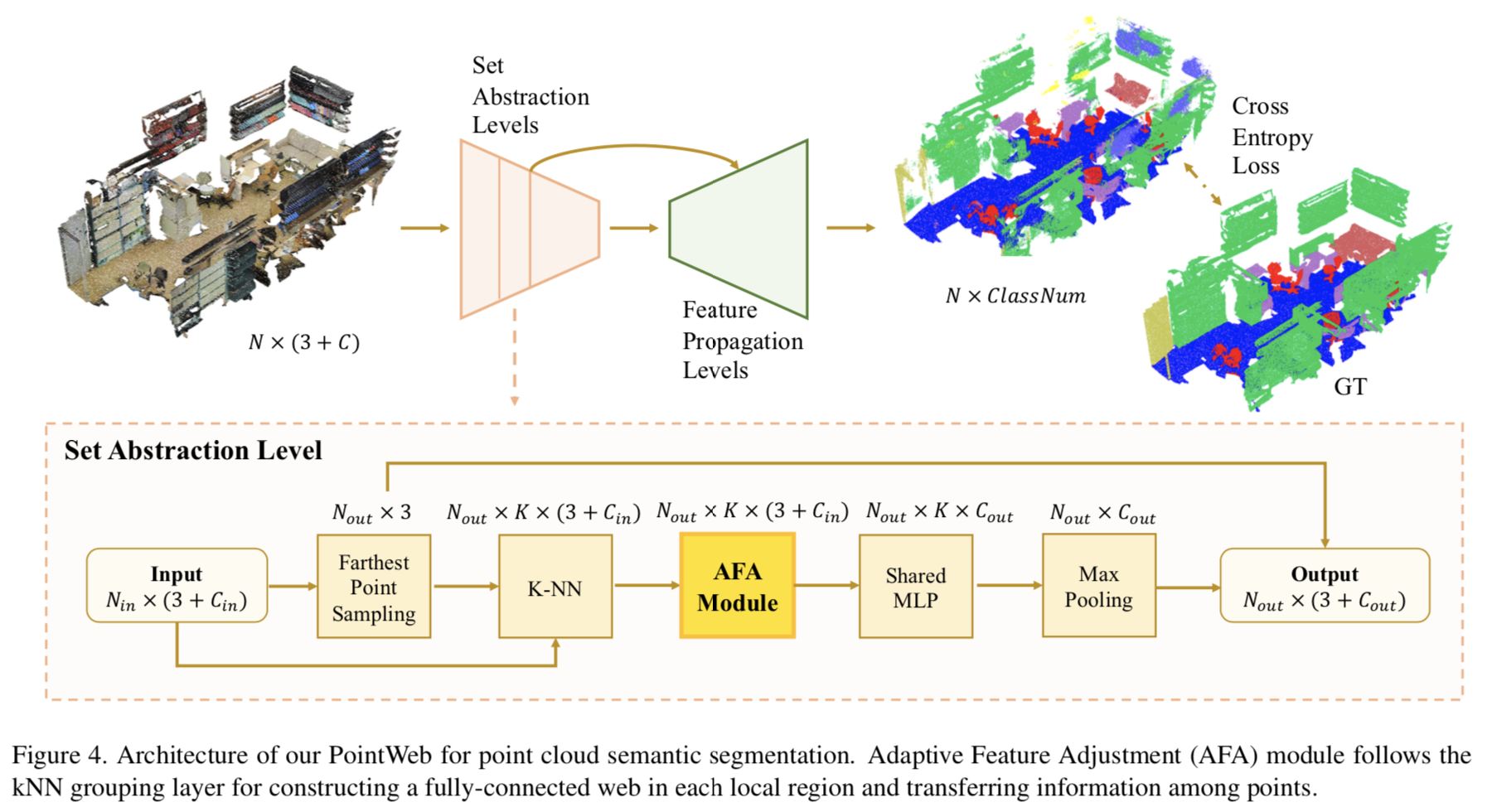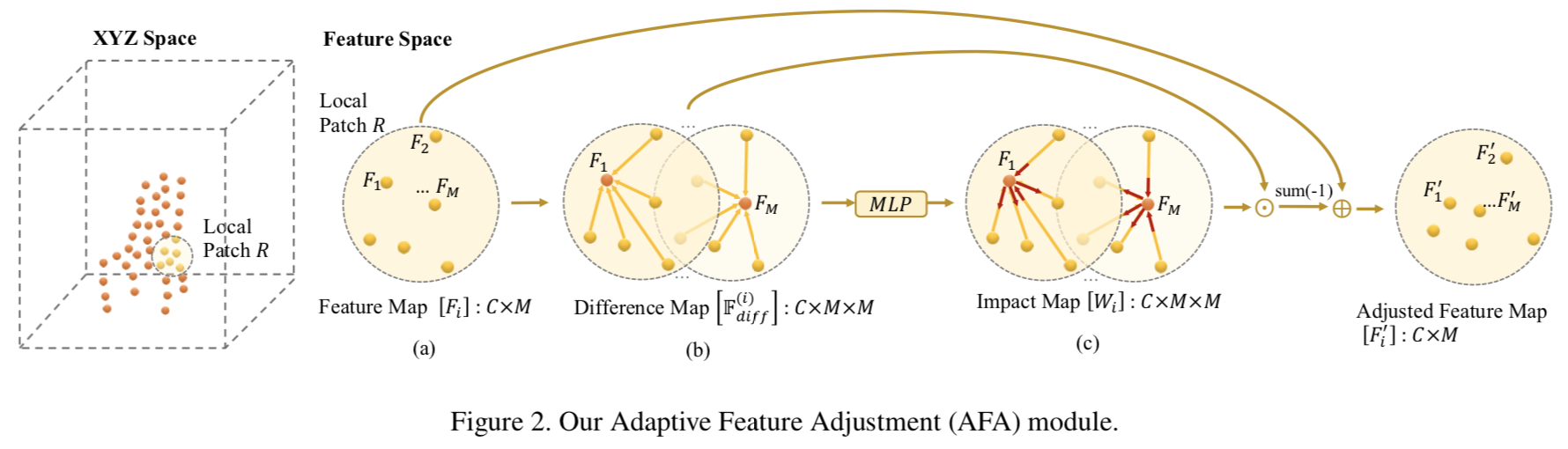AFA模块的演示图
1. 定义。

对于$R$域中的点，定义一个拥有$M$个点的点云集的特征为$\mathbb{F}=\{F_1, F_2,…,F_M\}$，其中$F_i \in {\reals}^C$,C是每一个点的特征的通道数(一般就是$xyz$三通道)。本文的目的就是获得一个这个集合的表达向量$F_{out} \in {\reals}^{C_{out}}$。PointNet++通过MLP和maxpooling来做，但是并没有考虑最开始点之间相互的影响。这篇论文则是将一个区域内的点都连接了起来，考虑他们之间的内在关系。这样可以获得邻域信息，增强局部表达能力。

2. AFA模块。

• 对于一个点$x_i$，首先先使用AFA模块来增强该点的特征，方式就是考虑其他点对该点特征的影响。可以把矫正后的特征$F’_i$ 定义为：

其中$\Delta F_i = f_{mod}(F_i, \mathbb{F}), \forall F_i \in \mathbb{F}$ ，也就是关键是找到这个$f_{mod}$，他计算了所有点对一个点的影响，也要注意，这个$F_i$也是包括在$\mathbb{F}$里边的。

• 本文核心就是自适应的学习$\mathbb{F}$ 对 $F_i$ 的的影响，表达为：

$f_{imp}$是学习到的在$F_j$和$F_i$之间的影响程度，而$f_{rel}$则是表示$F_j$ 如何影响 $F_i$ ，即到底是”拽过来”还是”推出去”。要注意到这个公式也包括了$F_i$对自己本身的影响。

• 定义$f_{imp}$。论文使用MLP来计算这一项。所以定义为：

其中$g$是一个用来combine $F_i$和 $F_j$的函数，用$w_{ij}$来表示$F_j$对$F_i$的影响因子。$g$的定义变成了关注点，直接拼合的话，一半儿的通道都是无用的；直接相加的话，就变成了一种对称状态，$F_i$给$F_j$的和$F_j$给$F_i$的变成了一样的，最终选择了$g(F_i, F_j)=F_i - F_j$，表示为两个特征之间的差值。特殊情况是当$i=j$时，$g(F_i, F_j)=F_i$。所以最终:

• 定义$f_{rel}$。一种方案是直接将得到的$w_{ij}$与$F_i$相乘，但是据实验证明效果不是最好的，我觉得可能因为直接相乘的话，变成了受$F_i$本身的影响方式，不是其他点给$i$的。随后，文章也确实换了一种，使用：

所以$f_{mod}$变成了：

• 最终的$F’_i$变成了：

• 以上的过程也可以用向量化来计算。对于第$i$个点来说，其影响因子map可表示为：

他是一个$C \times M$ 的矩阵，即$M$个点，每个$C$通道。那$f_{mod}$就可以表示为：

其中$\bigodot$是逐元素相乘，其中的${\mathbb{F}}^{(i)}_{diff}$定义为:

1. 带有局部特征模块的PointWeb

整体是嵌套在PointNet++上的。也就是作者觉得PointNet++对特征的聚合仅仅使用了max pooling，不是很靠谱，所以在提取特征前，Grouping layer后，加一个AFA模块。作者也说这项工作可以被看做是一个特征变换模块。

## 实验结果

accuracy avg.class accuracy overall
Relation-shape CNN 89.4 92.3

# KPConv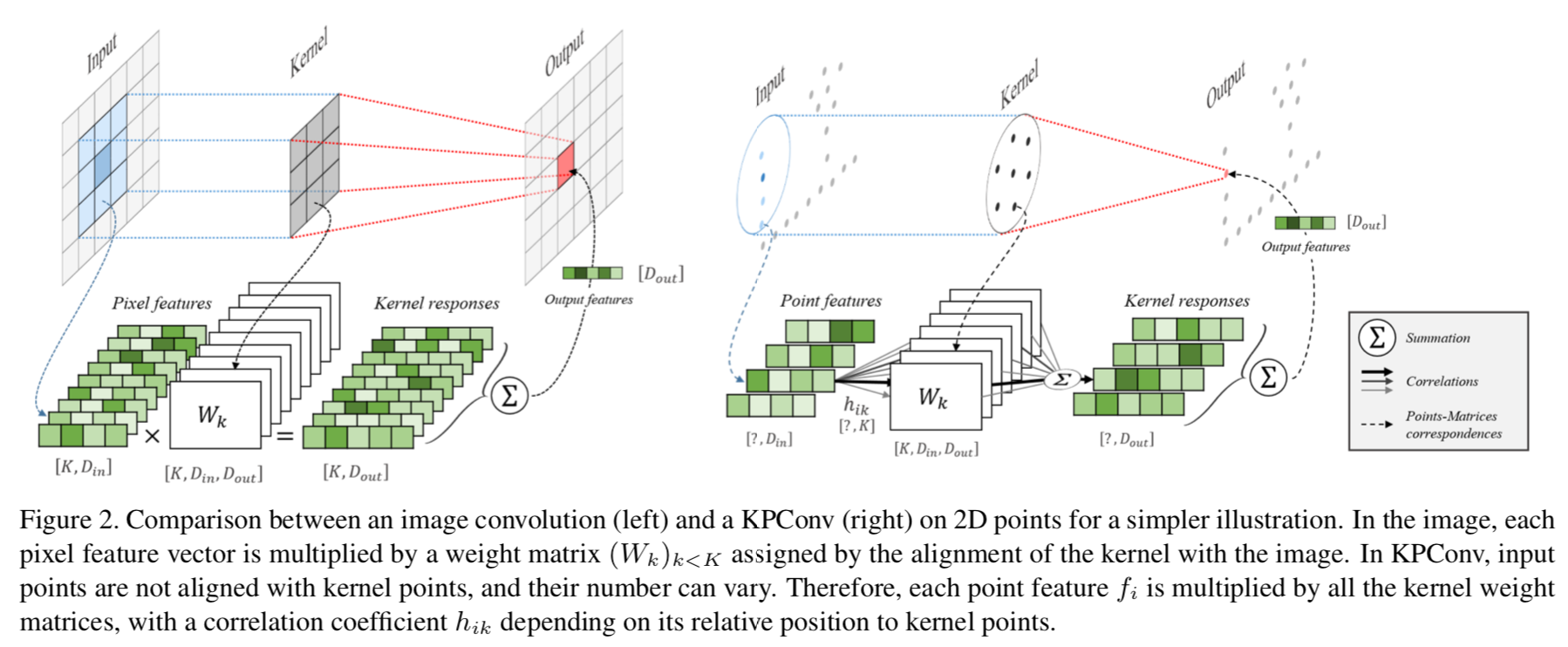## contributions：

• 提出一种新的点云卷积操作，叫做Kernel Point Convolution，即KPConv。概念来自于2D中的卷积，使用一系列的kernel points来定义在哪里应用核权重。
• 也同样提出了一种可变的卷积版本，通过学习局部的偏移来应用在kernel points上，这样在不同层产生不同的偏移，因此可以自适应在不同的层上。
• 同时为了适应点云的几何以及避免空的子集，还使用了Effective Receptive Field（ERF）来进行处理。

## 做法：

1. 点云中定义的核函数

如果说$x_i$与$f_i$分别是点集$\mathcal{P} \in {\mathbb{R}}^{N \times 3}$与特征集$\mathcal F \in {\mathbb{R}}^{N \times D}$中的一个点云与其对应的特征($N$个点的集合)。那对于点云$x$特征的通用的卷积可以定义为：

这意味着对于一个点$x$特征的卷积计算，是需要和其在一个子集内所有点的共同参与的。这里和二维不同的是，它先对一个点算出其在卷积过程中本身点的$C_in$个通道特征值与权重值的乘积结果，包括最后要变成几通道，然后全部$\mathcal{N}$ 集合中的向量再相加，得到最终$C_{out}$个通道的卷积值(二维里是先在单个通道求和3*3个值，然后求和$C_{in}$通道，再输出$C_{out}$组卷积核的结果，形成$C_{out}$个输出通道)

这篇论文同样也是以球体的方式划分点云变成若干子集。所以$\mathcal{N}_x=\{x_i \in \mathcal{P}||x_i-x|\le r\}$ ,也就是在一个半径为r的球体内取点。

此时，核心就是如何定义核函数$g$，在二维中他就是一个规则的矩阵，与被卷积区域逐点相乘再相加。在本文中，$g$的输入是以$x$为中心的邻域位置为输入，方便起见取$y_i=x_i-x$。

目的是想让$g$在在不同的区域给予不同的权重，这个区域有多种定义方式，最显而易见的就是直接用点去表示，因为很好定位。

因此，定义$\{\tilde{x}_k | k < K\} \subset \mathcal{B}_r^3$ 是核点云，以及$\{ W_k|k<K\} \subset \mathbb{R}^{D_{in} \times D_{out}}$ 是对应每一个点的权重矩阵。很好理解的是在二维中，一个像素点对应的其实也是卷积核中该位置全部拿出来后的一个矩阵，大小就是$D_{in} \times D_{out}$。

在论文中，作者将这个$g$定义为：

我觉得这里很巧妙的就是，二维中，一个点他就对应了卷积核中一个位置，直接相乘即可，但是因为三维中的无序，无法选定到底是哪一个，那作者就把所有点都进行了一下融合，作为一个点所对应的权重值，融合方式就是根据核点云中所有点到该点距离的远近来量化核点云不同点的影响。所以，这个$h$的定义就很容了，文中使用了$h(y_i, \tilde{x}_k)=max(0,1-\frac{|y_i-\tilde{x}_k|}{\sigma})$ 来衡量核点云中每个点的影响程度，其中$\sigma$是核点云的影响范围距离，根据输入的密度来选择。

2. 固定的和可变的核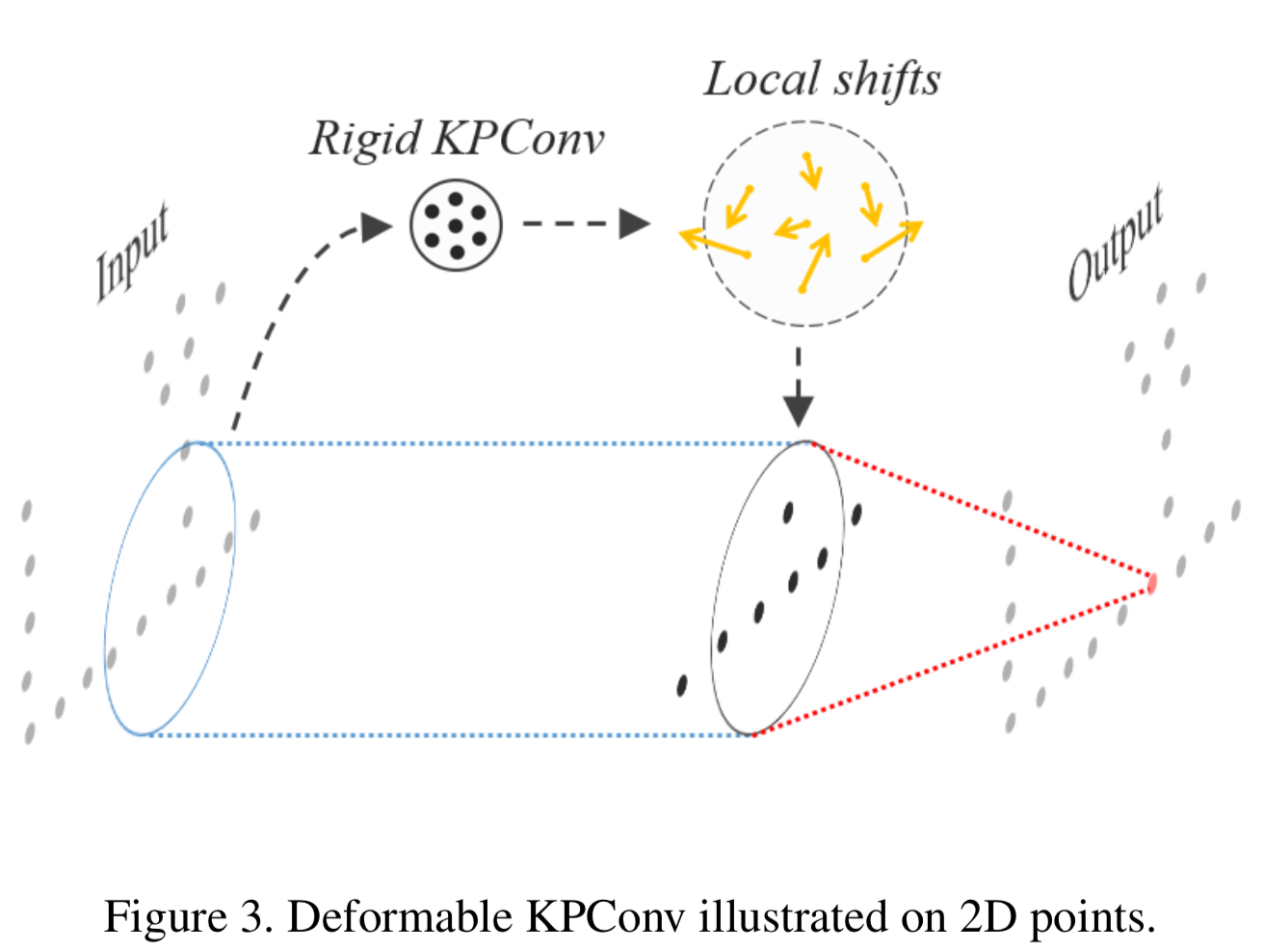可变卷积核在2D点云上的演示，注意那个Local shifts就是偏移

核点云的位置很重要。论文说如果选择固定(rigid)方式，那核点云的排列规则性需要设置好，为了满足不同的情况，最好的方式就是对于任意大小$K$个核点云，都可以可变自适应的排列。所以提出了deformable kernel的解决方案。

首先作者以一种让点相互排斥但是又组成一个球体的方式排列，选出其中一个点作为中心。设置这个球的半径为$1.5\sigma$，这样保证了点之间覆盖度一定的重叠。

既然每一层都是一个点集$\tilde{x}_k$，为了让他自己可变，作者干脆取一个所有层的并集，组成了${\tilde{x}_k}$，可以适用于每一个卷积层。作者也说，这种方式相对于一个合适的规则排布来说可能不会带来性能的提升。论文通过设置一个相对于每个要求的点$x$的偏移量$\Delta (x)$的方式来构成可变KPConv。所以改写原来的卷积方程为：

其中的$g_{deform}$可以定义为：

与之前的相比，其实就是对每个层的核点云位置进行了偏移。而这个偏移$\Delta _k(x)$论文中定义为了rigid Conv的一种输出，其计算方式就是将$D_{in}$ 映射到$3K$个值（$K$是核点云包含的点云数量），想想其实就是映射给每一个点云一个$xyz$的值，以此来作为偏移量。然后把这个偏移再加给rigid kernel每个点的位置$\tilde{x}_k$ 。就形成了一个可变的核点云。

之后，作者还加了几个正则化项，用来应对一些小问题。

## 实验结果：

accuracy avg.class accuracy overall
KPConv(rigid) - 92.9
KPConv(deform) - 92.7

# 五种方法的对比

model accuracy avg.class accuracy overall Feature extraction style
PointNet 86.2 89.2 MLP
PointNet++ - 90.7 MLP
PointNet++(with normal) - 91.9 MLP
RS-CNN - 93.6 MLP
PointWeb 89.4 92.2 MLP
KPConv(rigid) - 92.9 Kernel Point
KPConv(deform) - 92.7 Kernel Point